American Journal of Modern Physics
Volume 4, Issue 5, September 2015, Pages: 232-239

Characterization of Neutron Field in a Spherical Irradiation Facility

M. Tohamy1, M. Fayez-Hassan1, S. Abd El-Ghany2, S. M. El-Minyawi2, M. M. Abd El-Khalik1, M. N. H. Comsan1

1Experimental Nuclear Physics Department, Nuclear Research Centre, Atomic Energy Authority, Cairo, Egypt,

2Physics Department, Faculty of Science, Al-Azhar University (Girl's Branch), Cairo, Egypt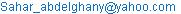(S. Abd El-Ghany)

M. Tohamy, M. Fayez-Hassan, S. Abd El-Ghany, S. M. El-Minyawi, M. M. Abd El-Khalik, M. N. H. Comsan. Characterization of Neutron Field in a Spherical Irradiation Facility. American Journal of Modern Physics. Vol. 4, No. 5, 2015, pp. 232-239. doi: 10.11648/j.ajmp.20150405.13

Abstract: A small-sized dual-hemisphere irradiation facility designed and constructed for using Am-Be isotopic neutron source to study and characterize the neutron field around sample irradiation position for obtaining maximum thermal neutron flux. The foil activation method based on 115In and 197Au was used for monitoring the thermal neutron flux. For field characterization, several combinations of moderation media have been tested (air, sand and water) aiming at the identification of the best combinational setups for sample irradiations using thermal neutrons. To account for epithermal contribution, reaction rates and flux calculations were corrected using tabulated values of Westcott g-factors and resonance integrals for these media. The optimal position for sample irradiation using thermal neutron for both water-water and water-sand setups, inside the irradiation channel, was found at about 6.9 to 10.9 cm from the center of the neutron source.

Keywords: Irradiation Facility, Am-Be Neutron Source, Source Shielding, Neutron House

Contents

1. Introduction

The neutron activation analysis (NAA) method is considered one of the most famous methods to investigate elements in a variety of sample matrices. In the NAA the sample to be analyzed is exposed to a thermal neutron ﬂux; then, the induced activity of the sample is measured using absolute or comparative method. For this purpose a previously described small-sized neutron irradiator prototype based on a 5 Ci (1.85×1011Bq) Am-Be isotopic neutron source was prepared . The use of the neutron irradiator presents the advantage of supplying a stable neutron ﬂux over a long period [2-4], thus eliminating the need for using expensive neutron producing devices such as reactors or accelerators. This way makes the analyzing process became agile, practical and economic. This work presents the optimization of the neutron irradiator for thermal and epithermal neutron production using several combinations of moderating media.

2. Experimental Facility Description

In this work, the neutron irradiation facility designed and constructed previously in the shape of a spherical container was used . The design aimed to achieve symmetry with respect to the position of the neutron source used at the center of the sphere. The facility was designed for the accommodation and safe-handling of the 5 Ci Am-Be isotopic neutron source. The neutron irradiator consists of a sphere of 60 cm in diameter made of a galvanized steel sheet and divided to two hemispheres. The lower hemisphere is an open to be used as container to be filled with different media. The upper hemisphere has a sealed bottom with embedded-in horizontal tube used to place the neutron source at the center of the sphere. Moreover, within the upper hemisphere a vertical cylindrical tube of 3.2 cm diameter was welded at the top of it and at the mid place of the source containing horizontal tube. The vertical tube is a devoted space for sample’s irradiation, and contains equally spaced holes of appropriate diameter to allow water or air to achieve homogeneous medium in the upper hemisphere.

The source contained in a stainless steel tube can be moved in the horizontal direction and its position is determined by the neutron source handling system. The experimental arrangement of the facility including the neutron irradiator, neutron house as well as the neutron source handling system is illustrated in Figure. 1. Measurement procedures are described in a previous paper .

A neutron house was used to accommodate the neutron source outside the facility . The shield of the neutron house was designed such that the dose rate at the outside surface of the shield did not exceed the dose limits given according to ICRP-60 .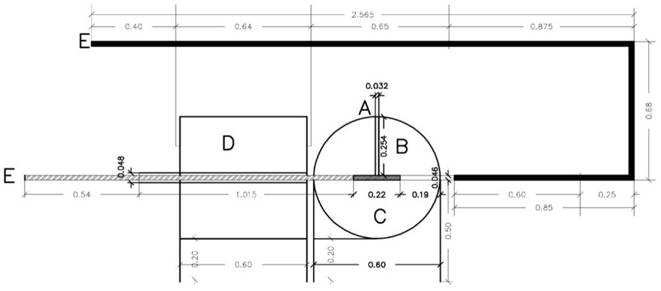Fig. 1. Experimental arrangement for the neutron flux measurements. A is the samples irradiation channel, B is the upper hemisphere, C is the lower hemisphere, D is the source housing, and E is the source handling system. All dimensions are in (m).

3. Experimental Procedures

The spectrometer used in this experiment was a HPGe coaxial detector of 70 % relative efficiency and 2.5 keV energy resolution at the 1332.5 keV g-ray of 60Co, coupled to associated electronics. The detected pulses were analyzed by an 8 k computerized multichannel analyzer. The Canberra’s software package Genie-2000 was used for recording the spectra and extraction of the necessary data from the spectra. Oak Ridge standard gamma point sources of 22Na, 57Co, 60Co, 133Ba, 137Cs and 152Eu were used for both the energy and efficiency calibrations of the system. The standard sources were positioned along the detector axis at distance 0, and 5 cm above the cap. To reduce the background effect, a cylindrical 5 cm thick lead-shield containing an inner concentric thin Cu cylinder was used.  Full-energy peak area was obtained and the absolute peak efficiency calculated using the following equation: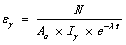(1)

where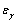is the full-energy peak efficiency, N is the corrected net peak area in counts/sec., Ao is the initial activity of standard source in Bq,  Iγ is the absolute gamma-ray intensity, λ is the decay constant, and  t  is the elapsed time since standardization. A typical plot for the log-log absolute efficiency as function of the gamma-ray energy is shown in Figure. 2 for the energy range of interest in our measurements. A second-order polynomial function was used to fit the plot.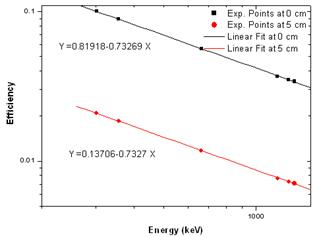Fig. 2. The log-log absolute efficiency fitting curve for 70% HPGe spectrometer measured at 0 and 5 cm distance.

In order to monitoring the thermal neutron flux by foil activation method, 115In and 197Au were irradiated. Five sample foils are fixed in a Plexiglas holder at distances 2.9, 6.9, 10.9, 14.9 and 18.9 cm far from the center of the neutron source in the samples channel A. The irradiation time (tir) was 68 hours in case of Au foils and 3 hours in case of In foils. When the foils are removed from the neutron field,  a suitable cooling times (tc) were employed, taking into consideration the half-lives of the elements of interest, after which the samples were counted using the HPGe spectrometer. The characteristic gamma-rays emitted from the activated foils were counted for a certain period of time (tm=15 min.). After counts have been logged, the data can be used to monitoring and calculate the flux at the foil during the experiment. Table I gives the physical parameters of the used foils. Table II shows the activation cross-section for the (n, γ) reaction of 115In and 197Au that yield radioactive products with convenient half-lives .

Table I. Physical parameters of samples used in the current experiments.

 Foils Group Size Purity (%) Thickness (μm) Weight (gm) In [C] 1´1 cm2 99.9 356.62 0.2596 Au* [A] 1´1 cm2 99.9 50.50 0.0976 Au* [B] 1´1 cm2 99.9 51.97 0.1004

* The gold samples are manufactured by Chempur, Feinchemikalien and Forschungs-bederf  Gmb H, Karlsruhe, Germany.

Table II. The activation properties of the used foils.

 Target Isotopic abundance (%) Reaction Decay mode (%) σact (barn) Half-life 115In 95.712 115In(n,γ)116mIn β-(100) 162.3 ±0.7 54.12 (min.) 197Au 100 197Au(n,γ)198Au β-(100) 98.8 ±0.3 64.6 (h.)

To calculate the thermal flux, two activity measurements were performed using bare and cadmium covered samples. The thickness of the cadmium cover was 480.0±0.5 μm, which has a high neutron absorption cross section for neutron energies below 0.4 eV. Among the obtained gamma spectra of the decay products the most intense gamma lines were chosen as shown in Table III .

Table III. The selected gamma lines emitted by 116mIn and 198Au.

 Target γ-Ray Energy Lines (keV) γ-Ray Intensity (%) 116mIn 416.86 27.7 1097.326 56.2 1293.558 84.4 198Au 411.802 96

4. Results and Discussions

4.1. Reaction Rate and Flux Calculation

The reaction rates (RR) were calculated for both Au and In samples using the well known formula as follows :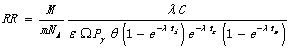(2)

where M is the atomic weight of the sample,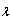is the decay constant,  C is the net counts, m is the sample weight, NA is the  Avogadro’s number,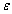is the detection efficiency,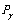is the gamma ray decay probability,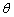is the isotopic abundance, ti,c,m  is  the irradiation, cooling and measuring time, respectively and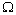is the detector solid angle given by:

Ω = ΩFd × ΩFS

Where, ΩFd =  1/2  is the foil detector solid angle and ΩFS is the foil source solid angle, calculated as,  ΩFS= area of the foil/(4π× (distance between foil and neutron source)2).

Equation (2) was used for both bare and cadmium (Cd) covered foils measurements.

The thermal RRth  was calculated as the difference between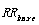and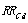,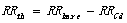(3)

The thermal and epithermal flux values were calculated using the following equations :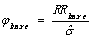(4)

Whereis the effective cross-section expressed as: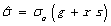(5)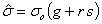where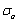is the 2200 m sec-1cross-section, g and s are functions of the temperature T and are measures of the departure of the cross-section law from the 1/v form in the thermal and epithermal regions, respectively, g is the Westcott g-factor, which equals 1.000 for Au and 1.022 for In foils , r is the epithermal index that varies from 0.011 to 0.047 depending on the irradiation facility used. For our facility r = 0.02 calculating according to the procedure described in reference . Furthermore, s is defined as: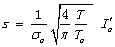(6)

Where, To =293.6 oK (20.5˚C), T is the irradiation facility temperature (300 oK), and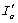is a reduced resonance integral which is obtained by subtracting the 1/v -term from the resonance integral Io and given by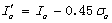for cadmium cutoff energy ECd of 0.5 eV.

On the other hand, the epithermal flux is given as: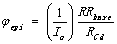(7)

where the resonance integral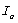taken as 1550 barn for Au and 2605 barn for In foils , and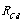is the cadmium ratio calculated according to equation:(8)

where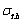= microscopic thermal neutron activation cross-section.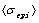= average epithermal neutron activation cross-section.

Finally, the thermal flux was obtained as: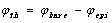(9)

The calculated value of reaction rate for thermal neutrons in the case of Au and In samples for the best configurationally setup (water-water) is shown in  Table IV.

Table IV. The calculated thermal neutron reaction  rate for both In and Au foils in case of water-water setup. The distance is center-to-center.

 Distance (cm) [In ] RRth (n.cm2/sec) [Au] RRth (n.cm2/sec) 416 (keV) 1097(keV) 1293(keV) 411 (keV) 2.9 2.76´10-16 3.52´10-16 3.21´10-16 3.35´10-16 6.9 1.63´10-15 2.07´10-15 1.89´10-15 2.25´10-15 10.9 2.33´10-15 2.87´10-15 2.62´10-15 3.16´10-15 14.9 2.03´10-15 2.53´10-15 3.30´10-15 2.75´10-15 18.9 1.36´10-15 1.73´10-15 1.58´10-15 1.93´10-15

4.2. Measured Neutron Fluxes

The thermal and epithermal fluxes were measured for different combinational setups to determine the best position for maximum thermal neutron flux for each setup[1,11]. The epithermal and thermal fluxes are calculated according to equations (7) and (9) for both In and Au samples, respectively.

The configurational setups were divided into two categories: one with the air in the upper hemisphere, and the second with the water in the upper hemisphere. The major difference is that condition for efficient neutron thermalization was satisfied in case of water (as abundant hydrogenous material) in the upper hemisphere as will be shown in the following sections. This is evident from the shape of the yield dependence curve characterized by neutron build up and decay. In previous work [1,11], in case of air in the upper hemisphere, the shape of the neutron flux curve dependence indicates that the condition for efficient thermalization was not yet satisfied (within the sample-source distance used in the current experiment), and hence the observed monotonic increase in the flux with distance.

4.2.1. Water-Air Setup

In this geometry we studied the effect of introducing hydrogenous material around the sample irradiation position. Water is known by its good thermalization properties, where the neutron diffusion length in water is 2.7 cm which means that even at such small distance, good efficient thermalization can be obtained. This reflects itself clearly in Figure 3 where the standard buildup/decay curve is observed.

4.2.2. Water-Sand Setup

In this setup we studied the effect of introducing dense media (sand) in the lower hemisphere on the neutron field around the irradiation position being in water. As is seen in Figure 4 noticeable increase in the neutron flux is observed. This may be attributed to the effect of the neutron reflection from the dense surface in the lower hemisphere. The results  show a characteristic neutron yield  buildup and decay with positions of maximum values ranging from 6.9 to 10.9 cm center-to-center distance.

4.2.3. Water-Water Setup

In this setup homogenous hydrogenous material (water) surrounds both the source and irradiation position. This setup is considered the most appropriate for obtaining efficient neutron thermalization. It is characterized by high thermal neutron flux and hence high induced activation in the samples. The obtained neutron yield dependences are presented in Figure 5. They show characteristic neutron yield buildup and decay with positions of maximum values ranging from 6.9 to 10.9 cm center-to-center distance. The decrease of the neutron yield following the maximum value indicates that at this source-sample distance the condition for thermal equilibrium was reached. The geometry setup is advantageous for measurements with thermal neutrons (analysis, cross-section).

In the previous setups, the data show that the maximum value for thermal neutron yield is obtained at the position 10.9 cm.

 [Au-samples] [In-samples]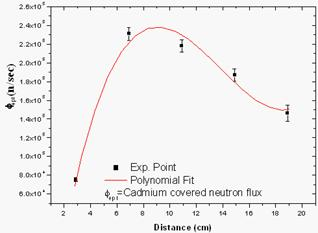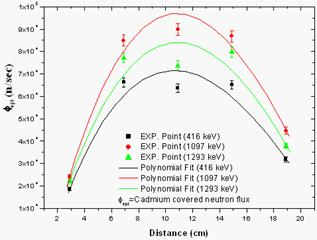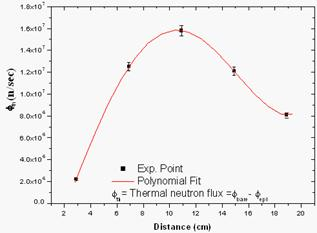Fig. 3. Total, epithermal and thermal neutron yield variation for Au and In samples versus distance from the center of the neutron source in water-air setup . Φ is corrected for a neutron build-up factor of 5.

 [Au-samples]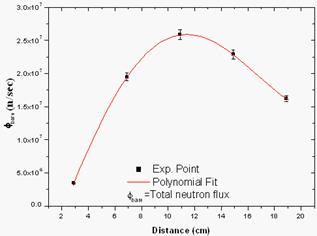[In-samples]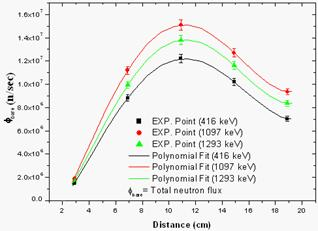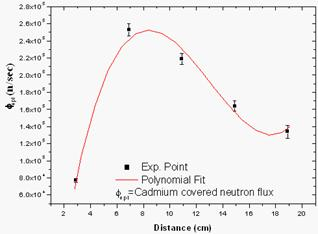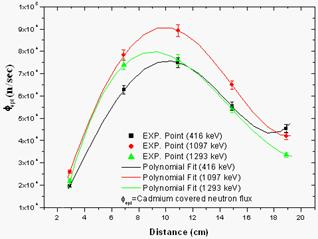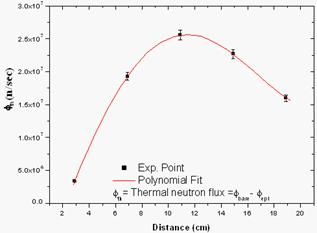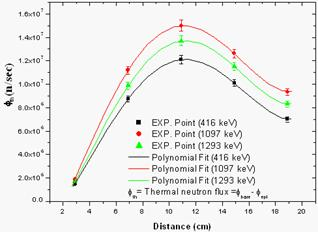Fig. 4. Total, epithermal and thermal neutron yield variation for Au and In samples versus distance from the center of the neutron source in water-sand setup . Φ is corrected for a neutron build-up factor of 5.

 [Au-samples][In-samples]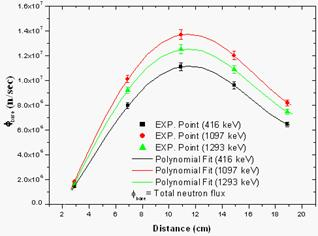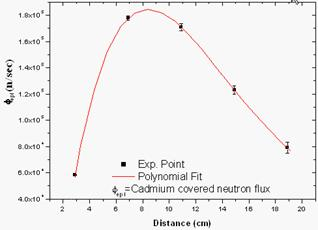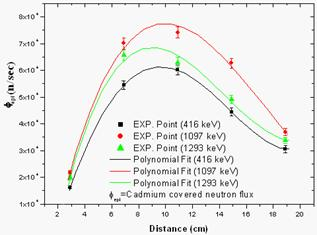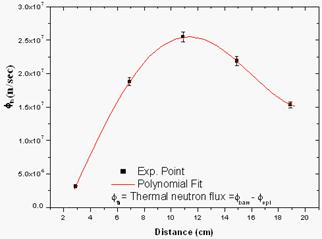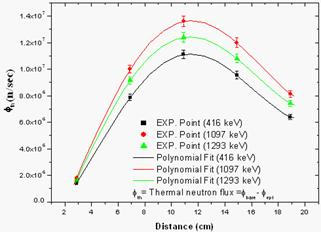Fig. 5. Total, epithermal and thermal neutron yield  variation for Au and In samples versus distance from the center of the neutron source in water-water setup .Φ is corrected for a neutron build-up factor of 5.

5. Conclusion

In this study a small-sized spherical irradiator consisting of two hemispheres has been designed and constructed for the aim of optimizing neutron field for neutron activation using thermal neutrons from an isotopic Am-Be neutron source. Additionally, for the safe source storage and handling, a neutron house is provided for the constructed irradiator prototype [1,11]. TT The neutron field characterization inside the irradiator was performed using the foil activation technique with both Indium and Gold foils.

Three combinational setups have been presented, namely: water-air, water-sand and water-water setup for study the thermal neutron irradiations. To account for epithermal contribution, reaction rates and flux calculations were corrected using both Westcott g-factors and resonance integrals for these setups.

It was found that the water-water and water-sand setups are the most suitable setups for subcadmium (thermal neutron yield) irradiation at distance varying from 6.9 to 10.9 cm where the thermal neutron yield is the maximum. For these setups at a distance 10.9 cm both activation foils (In and Au) give an average value of  (1.66±0.05)´107 n/sec in case of water-sand setup and (1.85±0.65)´107 n/sec in case of water-water setup.

Acknowledgment

This work was carried as collaboration between Academy of Scientific Research and Technology, Experimental Nuclear Physics Department, Nuclear Research Centre, Atomic Energy Authority and the Faculty of Science, Girls Branch, Al-Azhar University.

The technician teams of the Nuclear Reactions Unit at Inshas are particularly acknowledged.

References

1. M. Tohamy, M. Fayez-Hassan, S. Abd El-Ghany, S.M. El-Minyawi, M.M. Abd El-Khalik and M.N.H. Comsan, "A Dual-Hemisphere Irradiation Facility for 241Am-Be Isotopic Neutron Source", Journal of Nuclear and Radiation Physics, Vol. 5 No. 1&2, 51, (2010), http://www.physicsegypt.org/jnrp/
2. R. B. M. Sogbadji, B. J. B. Nyarko, E. H. K. Akaho, R. G. Abrefah, Determination of Neutron Fluxes and Spectrum Shaping Factors in Irradiation Sites of Ghana’s Miniature Neutron Source Reactor (mnsr) by Activation Method after Compensation of Loss of Excess Reactivity, World Journal of Nuclear Science and Technology, 1, pp. 50-56, 2011.
3. Parisa Akhlaghi, Laleh Rafat-Motavalli and Seyed Hashem Miri-Hkimabad, The measurements of thermal neutron flux distribution in a paraffin phantom, Pramana  journal of physics, Vol. 80, No. 5, pp. 873–885, 2013
4. E. Mensimah, R.G. Abrefah, B.J.B. Nyarko, J.J. Fletcher, M. Asamoah, Neutron flux determination in irradiation sites of an Am–Be neutron source at NNRI, Annals of Nuclear Energy, Vol. 38, pp. 2303–2308, 2011.
5. ICRP, Recommendations of the International Commission on Radiological Protection. ICRP-60, (1991).
6. "Handbook on Nuclear Activation Data", IAEA, Technical Report Series No.273, Vienna, (1987).
7. I. S. Grigoriev, and E. Z. Meilikhov, (Eds.),"Handbook of Physical Quantities", Moscow, Russia, CRC Press, (1997).
8. C. H. Westcott, "Effective Cross Section Values for Well-Moderated Thermal Reactor Spectra", AECL-110, (1960).
9. E. Gryntkis, D. E. Cullen and G. Mundy, "Thermal neutron cross-section and infinite dilution Resonance integrals" as a part of reference.
10. S.F. Mughabghab, M. Divadeenam, and N. E. Holden, "Neutron Cross Sections (Vol.1), Neutron Resonance Parameters and Thermal Cross Sections", Academic Press, New York, (1981).
11. M. Tohamy, "Optimization of Neutron Field in Neutron Irradiation Facility" M.Sc. thesis, Faculty of Science, Al-Azhar University, 2009.

 Contents 1. 2. 3. 4. 4.1. 4.2. 5.
Article ToolsAbstractPDF(415K)